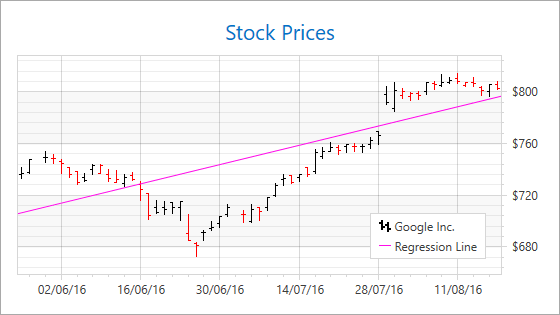# Regression Lines

A Regression Line is a series indicator that allows you to perform linear regression analysis over series points. The analysis aims to find a line that is as close to all series’s points as possible using the method of least squares to determine the underlying line’s equation.## How to Add a Regression Line to a Chart

The following markup shows how to build a regression line based on a stock series‘s High values:

``````<dxc:StockSeries2D.Indicators>
<dxc:RegressionLine ValueLevel="High"
ShowInLegend="True"
LegendText="Regression Line"/>
</dxc:StockSeries2D.Indicators>
``````

The code above uses the following classes and properties:

Class or Property Description
XYSeries2D.Indicators The series indicators’ collection.
RegressionLine The regression line.
RegressionLine.ValueLevel Specifies a point value used to build a regression line.
Indicator.ShowInLegend Specifies whether to show a regression line in the legend.
Indicator.LegendText Specifies the text that indicates the indicator in the legend.